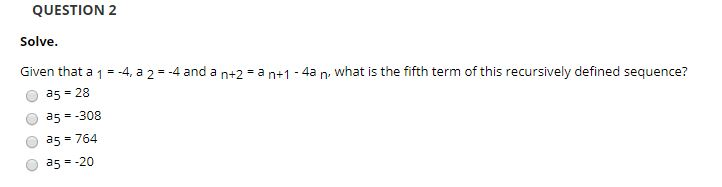# QUESTION 2Solve.Given that a 1 = -4, a 2 = -4 and awhat is the fifth term of this recursively defined sequence?n+2 = a n+14an.a5 = 28a5 = -308a5 = 764a5 = -20

Question
8 viewshelp_outlineImage TranscriptioncloseQUESTION 2 Solve. Given that a 1 = -4, a 2 = -4 and a what is the fifth term of this recursively defined sequence? n+2 = a n+1 4a n. a5 = 28 a5 = -308 a5 = 764 a5 = -20 fullscreen
check_circle

Step 1

Given

Step 2
Step 3

### Want to see the full answer?

See Solution

#### Want to see this answer and more?

Solutions are written by subject experts who are available 24/7. Questions are typically answered within 1 hour.*

See Solution
*Response times may vary by subject and question.
Tagged in

### Math